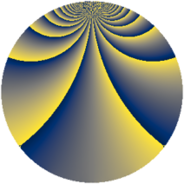Properties

 Label 1840.1.bqLevel $1840$ Weight $1$ Character orbit 1840.bq Rep. character $\chi_{1840}(239,\cdot)$ Character field $\Q(\zeta_{22})$ Dimension $20$ Newform subspaces $1$ Sturm bound $288$ Trace bound $0$

Related objects

Defining parameters

 Level: $$N$$ $$=$$ $$1840 = 2^{4} \cdot 5 \cdot 23$$ Weight: $$k$$ $$=$$ $$1$$ Character orbit: $$[\chi]$$ $$=$$ 1840.bq (of order $$22$$ and degree $$10$$) Character conductor: $$\operatorname{cond}(\chi)$$ $$=$$ $$460$$ Character field: $$\Q(\zeta_{22})$$ Newform subspaces: $$1$$ Sturm bound: $$288$$ Trace bound: $$0$$

Dimensions

The following table gives the dimensions of various subspaces of $$M_{1}(1840, [\chi])$$.

Total New Old
Modular forms 200 20 180
Cusp forms 80 20 60
Eisenstein series 120 0 120

The following table gives the dimensions of subspaces with specified projective image type.

$$D_n$$ $$A_4$$ $$S_4$$ $$A_5$$
Dimension 20 0 0 0

Trace form

 $$20 q + 2 q^{5} + 2 q^{9} + O(q^{10})$$ $$20 q + 2 q^{5} + 2 q^{9} - 2 q^{25} - 4 q^{29} + 4 q^{41} - 24 q^{45} - 20 q^{49} + 4 q^{61} - 2 q^{81} - 4 q^{89} + O(q^{100})$$

Decomposition of $$S_{1}^{\mathrm{new}}(1840, [\chi])$$ into newform subspaces

Label Dim. $$A$$ Field Image CM RM Traces $q$-expansion
$a_{2}$ $a_{3}$ $a_{5}$ $a_{7}$
1840.1.bq.a $20$ $0.918$ $$\Q(\zeta_{44})$$ $D_{22}$ $$\Q(\sqrt{-5})$$ None $$0$$ $$0$$ $$2$$ $$0$$ $$q+(-\zeta_{44}^{7}-\zeta_{44}^{9})q^{3}+\zeta_{44}^{6}q^{5}+\cdots$$

Decomposition of $$S_{1}^{\mathrm{old}}(1840, [\chi])$$ into lower level spaces

$$S_{1}^{\mathrm{old}}(1840, [\chi]) \cong$$ $$S_{1}^{\mathrm{new}}(460, [\chi])$$$$^{\oplus 3}$$